# Exercise E11.1 Constructions NCERT Solutions Class 9

## Chapter 11 Ex.11.1 Question 1

Construct an angle of $$90^\circ$$ at the initial point of a given ray and justify the construction.

### Solution

Reasoning:

We need to construct two adjacent angles each of $$60$$ degrees and bisect the second one to construct $$90$$ degree.

Steps of Construction: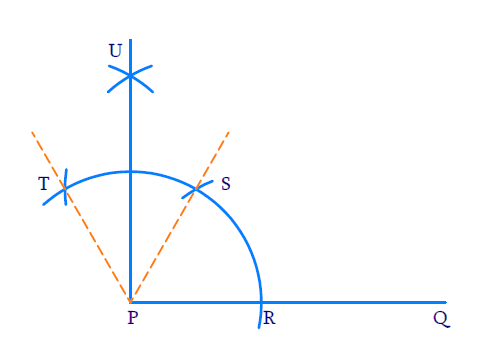(i) Draw ray $$PQ$$

(ii) To construct $$60^{\circ}$$ angle. Draw an arc of any radius with $$P$$ as center intersecting $$PQ$$ at $$R$$ with $$R$$ as center and same radius draw an arc intersecting the previous arc at $$S$$. \begin{align}\angle {SPQ}=60^{\circ}\end{align}

(iii) To construct adjacent $$60^{\circ}$$ angle. With $$S$$ as the center and same radius as before intersecting the initial arc at $$T$$ $$\angle {TPS}$$ will be $$60^\circ$$

(iv) To bisect $$\angle {TPS} :$$

With $$T$$ and $$S$$ as centers and same radius as before draw two arcs to intersect each other at $$U$$. \begin{align}\angle {UPS}=\frac{1}{2} \angle {TPS}=30^{\circ}\end{align}

(v)  Join $$P$$ and $$U$$ to get an angle of $$60^{0}$$ at initial point $$P$$.

\begin{align} \angle {UPQ} &=\angle {UPS}+\angle {SPR} \\ &=30^{\circ}+60^{\circ} \\ &=90^{\circ} \end{align}

## Chapter 11 Ex.11.1 Question 2

Construct an angle of $$45^\circ$$ at the initial point of a given ray and justify the construction.

### Solution

Reasoning:

We need to construct two adjacent angles each of $$60^\circ$$ degrees and bisect the second one to construct $$90^\circ$$ . Then bisect the $$90^\circ$$ angle to get $$45^\circ$$

\begin{align}\frac{60^{0}+\frac{60^{0}}{2}}{2}=45^{0}\end{align}

Steps of Construction: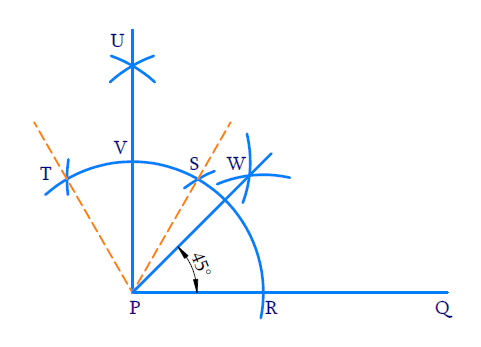(i) Draw ray $$PQ$$.

(ii) To construct an angle of $$60^{o}$$ . With $$P$$ as center draw a wide arc of any radius to intersect the ray at $$R$$. With $$R$$ as center and same radius draw an arc to intersect the initial one at $$S$$. $$\angle {SPR}=60^{\circ}$$ .

(iii) To construct adjacent angle of $$60^{\circ}$$ .

With $$S$$ as centre and same radius draw an arc to intersect the previous arc at $$T$$

$\angle {TPS}=60^{\circ}$

(iv) To bisect $$\angle {TPS}$$

With $$T$$ and $$S$$ as centre and same radius, draw arcs to intersect each other at $$U$$.

\begin{align}\angle {UPS}=\frac{1}{2} \angle {TPS}=30^{\circ}\end{align}

(v) Join $$P$$ and $$U$$ to intersect the initial arc at $$V$$.

\begin{align} \angle {UPQ} &=\angle {UPS}+\angle {SPR} \\ &=30^{\circ}+60^{\circ} \\ &=90^{\circ} \end{align}

(vi) To bisect $$\angle {UPQ}$$

With $$R$$ and $$V$$ as centers and radius greater than half of $$RV$$, draw arc to intersect each other at $$W$$. Join $$PW$$. $$PW$$ is the angle bisector of $$\angle U P Q$$

\begin{align} \angle {WPQ} &=\frac{1}{2} \angle {UPQ} \\ &=\frac{1}{2} \times 90^{\circ} \\ &=45^{\circ} \end{align}

(vii) Ray $$PW$$ forms an angle of $$45^{\circ}$$ with ray $$PQ$$ at the initial point.

## Chapter 11 Ex.11.1 Question 3

Construct the angles of the following measurements:

(i) $$30^{\circ}$$

(ii) $$22 \frac{1}{2}^{\circ}$$

(iii) $$15^{\circ}$$

### Solution

$${\rm{(i)}}\;\;30^{\circ}$$

Reasoning:

We need to construct an angle of $$60$$ degrees and then bisect it to get an angle measuring $$30^\circ$$

Steps of Construction: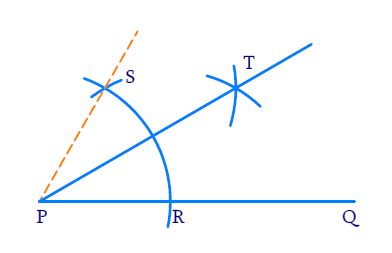(i) Draw ray $$PQ$$.

(ii) To construct an angle of $$60^{\circ}$$ .

With $$P$$ as centre and any radius, draw a wide arc to intersect $$PQ$$ at $$R$$. With $$R$$ as centre and same radius draw an arc to intersect the initial arc at $$S$$. $$\angle {SPR}=60^{\circ}$$

(iii) To bisect $$\angle {SPR}$$

With $$R$$ and $$S$$ as centres and same radius draw two arcs to intersect at $$T$$. Join $$P$$ and $$T$$ i.e. $$PT$$ is the angle bisector. Hence,

\begin{align}\angle {TPR}=\frac{1}{2} \angle {SPR}=30^{\circ}\end{align}

$${\rm{(ii)}}\;\;22 \frac{1}{2}^{\circ}$$

Reasoning:

We need to construct two adjacent angles of and bisect the second one to get a angle. This has to be bisected again to get a $$45^\circ$$ angle. The $$45^\circ$$ angle has to be further bisected to get \begin{align}22 \frac{1}{2}^{\circ}\end{align} angle.

\begin{align} 22 \frac{1}{2}^{\circ} &=\frac{45^{\circ}}{2} \\ 45^{\circ} &=\frac{90^{\circ}}{2}=\frac{30^{\circ}+60^{\circ}}{2} \end{align}

Steps of Construction: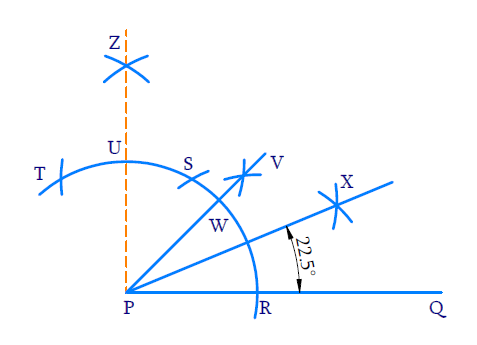(i) Draw ray $$PQ$$

(ii) To construct an angle of $$60^{\circ}$$

With $$P$$ as center and any radius draw a wide arc to intersect $$PQ$$ at $$R$$. With $$R$$ as center and same radius draw an arc to intersect the initial arc at $$S$$. $$\angle {SPR}=60^{\circ}$$

(iii)To construct adjacent angle of $$60^{\circ}$$ .

With $$S$$ as the center and same radius as before, draw an arc to intersect the initial arc at $$T$$ $$\angle {TPS}=60^{\circ}$$ .

(iv)To bisect $$\angle {TPS}$$

With $$T$$ and $$S$$ as centers and same radius as before, draw arcs to intersect each other at $$Z$$ Join $$P$$ and $$Z$$ $$\angle {ZPQ}=90^{\circ}$$

(v) To bisect $$\angle {ZPQ}$$

With $$R$$ and $$U$$ as centers and radius than half of $$RU$$, draw arcs to intersect each other at $$V$$. Join $$P$$ and $$V$$. $$\angle {VPQ}=45^{0}$$

(vi) To bisect $$\angle {VPQ}=45^{0}$$

With $$W$$ and $$R$$ as centers and radius greater than half of $$WR$$, draw arcs to intersect each other at $$X$$. Join $$P$$ and $$X$$. $$PX$$ bisects $$\angle {VPQ}$$

Hence,

\begin{align} \angle {XPQ} &=\frac{1}{2} \angle {WPQ} \\ &=\frac{1}{2} \times 45^{0} \\ &=22 \frac{1}{2} \end{align}

$${\rm{(iii)}}\;\;15^{\circ}$$

Reasoning:

We need to construct an angle of 60 degrees and then bisect it to get an angle measuring $$30^\circ$$. This has to be bisected again to get a $$15^\circ$$ angle.

\begin{align}15^{0}=\frac{30^{\circ}}{2}=\frac{\frac{60^{0}}{2}}{2}\end{align}

Steps of Construction: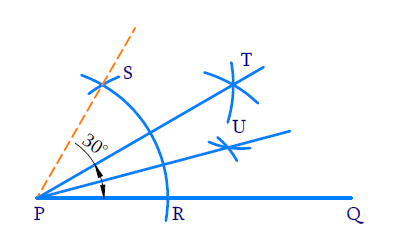(i) Draw ray $$PQ$$.

(ii) To construct an angle of $$60^{\circ}$$ .

With $$P$$ as center and any radius draw a wide arc to intersect $$PQ$$ at $$R$$. With $$R$$ as center and same radius draw an arc to intersect the initial arc at $$S$$. $$\angle {SPR}=60^{\circ}$$

(iii) Bisect $$\angle {SPR}$$ . With $$R$$ and $$S$$ as centers and radius greater than half of $$RS$$ draw arcs to intersect each other at $$T$$. Join $$P$$ and $$T$$ i.e. $$PT$$ is the angle bisector of $$\angle {SPR}$$ .

\begin{align} \angle {TPQ} &=\frac{1}{2} \angle{SPR} \\ &=\frac{1}{2} \times 60^{\circ} \\ &=30^{\circ} \end{align}

(iv)To bisect $$\angle {TPQ}$$

With $$R$$ and $$W$$ as centers and radius greater than half of $$RT$$, draw arcs to intersect each other at $$U$$ Join $$P$$ and $$U$$. $$PU$$ is the angle bisector of $$\angle {TPQ}$$ .

\begin{align}\angle {UPQ}=\frac{1}{2} \angle {TPQ}=15^{\circ}\end{align}

## Chapter 11 Ex.11.1 Question 4

Construct the following angles and verify by measuring them by a protractor:

(i) $$75^{\circ}$$

(ii) $$105^{\circ}$$

(iii) $$135^{\circ}$$

### Solution

$${\rm{(i)}}\;\;75^{\circ}$$

Reasoning:

We need to construct two adjacent angles of $$60^{\circ}$$.The second angle should be bisected twice to get a $$15^{\circ}$$ angle.

\begin{align}75^{\circ}&=60^{\circ}+15^{\circ} \\ 15^{\circ}&=\frac{30^{\circ}}{2}=\frac{60^{\circ}}{2} \div \frac{1}{2}\end{align}

Steps of Construction: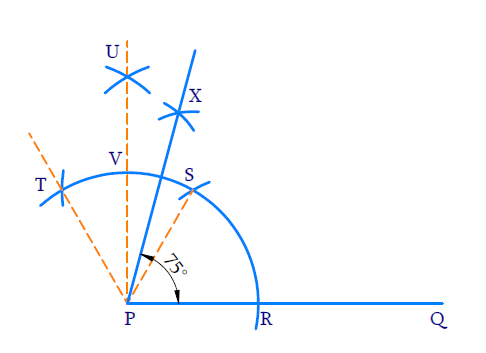(i) Draw ray $$PQ$$.

(ii) To construct an angle of $$60^{\circ}$$ .

With $$P$$ as centre and any radius draw a wide arc to intersect $$PQ$$ at $$R$$ With $$R$$ as centre and same radius draw an arc to intersect the initial arc at $$S$$ .$$\angle \mathrm{SPR}=60^{\circ}$$

(iii) To construct adjacent angle of $$60^{\circ}$$ with $$S$$ as center and same radius draw an arc to $$T$$ intersect the initial arc.

(iv) To bisect $$\angle \mathrm{SPT}$$

With $$T$$ and $$S$$ as centres and same radius draw arcs to bisect each other at $$U$$ Join $$U$$ and $$P$$. \begin{align}\angle \mathrm{UPS}=\frac{1}{2} \angle \mathrm{TPS}=30^{\circ}\end{align}

(v) To bisect $$\angle \mathrm{UPS}$$

With $$V$$ and $$S$$ as centers and radius greater than half of $$VS$$ draw arcs to intersect each other at $$X$$

\begin{align} \angle {XPS} &=\frac{1}{2} \angle {UPS} \\ &=\frac{1}{2} \times 30^{0} \\ &=15^{\circ} \\ \angle {XPQ} &=\angle {XPS}+\angle {SPQ} \\ &=15^{\circ}+60^{\circ} \end{align}

$${\rm{(ii)}}\;\;105^{\circ}$$

Reasoning:

We need to construct two adjacent angles of $$60^{\circ}$$ . In the second angle we need to bisect it to get two $$30^{\circ}$$ angles. The second $$30^{\circ}$$ angle should be bisected again to get a $$15^{\circ}$$ angle. Together we can make an angle of $$105^{\circ}$$ .

\begin{align} 105^{\circ} &=60^{\circ}+45^{\circ} \\ 45^{\circ} &=60^{\circ}+30^{\circ}+15^{\circ} \end{align}

Steps of Construction: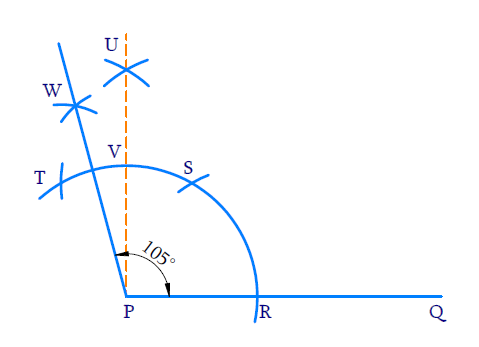(i) Draw ray $$PQ$$

(ii) To construct an angle of $$60^{\circ}$$.

With $$P$$ as centre and any radius draw a wide arc to intersect $$PQ$$ at $$R$$. With $$R$$ as centre and same radius draw an arc to intersect the initial arc at $$S$$. $$\angle \mathrm{SPR}=60^{\circ}$$

(iii) To construct an adjacent angle of $$60^{\circ}$$ with $$S$$ as the center and same radius as before draw an arc to intersect the initial arc at $$T$$. $$\angle \mathrm{TPS}=60^{\circ}$$

(iv) To bisect $$\angle \mathrm{TPS}$$

With $$T$$ and $$S$$ as centres and same radius draw arcs to bisect each other at $$U$$. Join $$U$$ and $$P$$

\begin{align}\angle \text{UPS = }\frac{1}{2}\angle \text{TPS }=\frac{1}{2}\times {{60}^{0}}\text{=30}{}^\circ \end{align}

(v)To bisect $$\angle \text{UPT}$$

With $$T$$ and $$V$$ as centers and radius greater than half of $$TV,$$draw arcs to intersect each other at $$W$$. Join $$P$$ and $$W$$.

\begin{align} \angle {WPU} &=\frac{1}{2} \angle {UTP} \\ &=\frac{1}{2} \times 30^{\circ} \\ &=15^{\circ} \\ \angle {WPR} &=\angle {WPU}+\angle {UPS}+\angle {SPR} \\ &=15^{\circ}+30^{\circ}+60^{\circ} \\ &=105^{\circ} \end{align}

$${\rm{(iii)}}\;\;135^{\circ}$$

Reasoning:

We need to construct three adjacent angles of $$60^{\circ}$$ each. The third angle should be bisected twice successively to get an angle of $$15^{\circ}$$ . Together we will get an angle of $$135^{\circ}$$ .

\begin{align} 135^{\circ} &=15^{\circ}+60^{\circ}+60^{\circ} \\ 15^{\circ} &=\frac{60^{\circ}}{2} \div \frac{1}{2} \end{align}

Steps of Construction: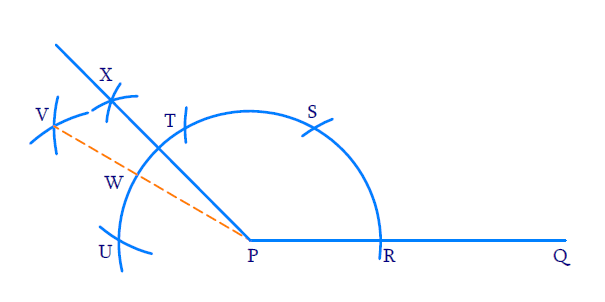(i) Draw ray$$PQ$$

(ii) To construct an angle of $$60^{\circ}$$ .

With $$P$$ as the center and any radius draw an arc to intersect $$PQ$$ at $$R$$.$$\angle \mathrm{SPR}=60^{\circ}$$

(iii) To construct adjacent angle of $$60^{\circ}$$

With $$S$$ as the center and same radius as before draw an arc to intersect the initial arc at $$T$$. $$\angle \mathrm{TPS}=60^{\circ}$$

(iv) To construct the second adjacent angle of $$60^{\circ}$$

With $$T$$ as centre and some radius as before draw an arc to intersect the initial arc at $$U$$

$\angle \mathrm{UPT}=60^{\circ}$

(v) To bisect $$\angle \mathrm{UPT}$$

With $$T$$ and $$U$$ as centers and same radius as before draw an arc to intersect each other at $$V$$ $$\angle \mathrm{VPT}=\angle \mathrm{VPU}=30^{\circ}$$

(vi) To bisect $$\angle \mathrm{VPT}$$

With $$W$$ and $$T$$ as centers and radius greater than half of $$WT$$ draw arcs to intersect each other at $$X$$.

\begin{align}XPT&=\angle XPV \\&=7\frac{1}{2}\angle V\text{PT}\\&=\frac{1}{2}\times {{30}^\circ}=\,15{}^\circ \\ \\ \angle XPQ &= \angle \text{XPT + }\angle TPS + \angle SPR \\&= 15{}^\circ + 60{}^\circ + 60{}^\circ \\&=135{}^\circ \,\,\, \\\end{align}

## Chapter 11 Ex.11.1 Question 5

Construct an equilateral triangle, given its side and justify the construction.

### Solution

Reasoning:

An equilateral triangle has three equal sides and three angles equal to $$60^\circ$$.We know how to construct an angle of $$60^{\circ}$$ .

Steps of Construction: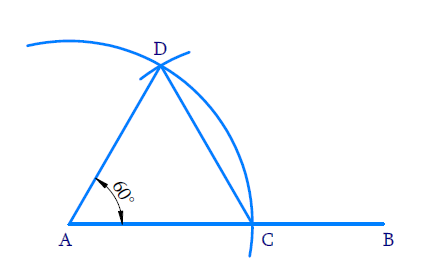(i) Draw ray $$AB$$

(ii) With $$A$$ as center and radius equal to $$3\;\rm{cm}$$ draw an arc to cut ray $$AB$$ at $$C$$ such that $$AC= 3\;\rm{cm}$$

(iii) With $$C$$ as the center and radius equal to $$AC$$, draw an arc to intersect the initial arc at $$D$$.

(iv) Join $$AD$$ and $$AC$$.

Thus,Triangle $$ADC$$ is an equilateral triangle.

Justification:

$$\rm{AC = AD}$$ (By construction since the radius of the arc is the same)

$$\rm{AC = CD}$$  (By construction since the same radius was used again)

$$\therefore \rm{AC = AD = CD}$$

$$\therefore ADC$$ is an equilateral triangle

Instant doubt clearing with Cuemath Advanced Math Program